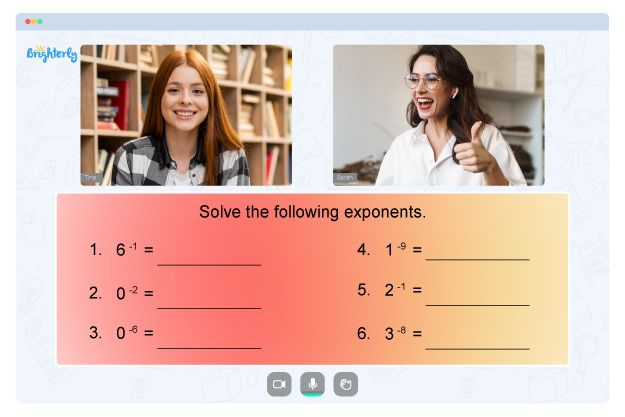Mathematical education is incomplete without the knowledge of probability since applied mathematics relies heavily on the concept. Unfortunately, many students still have difficulties understanding and applying the concept to everyday scenarios.

Is your youngster trying to understand the topic of probability? Here is everything you should know about 7th grade probability worksheets.

Before we start

7th-grade math is something that throws many parents off balance. Math topics at this stage are tricky, not every parent can help their kid easily, even if they want to. 7th-grade math is considered challenging by teachers, tutors, and parents alike.

If you want your child to tackle 7th-grade math quickly and worry-free, you should consider Brighterly’s professional tutors. Sign up for a free demo lesson.

## Why Do Brighterly Tutors Use 7th Grade Probability Worksheets?

Brighterly is an online platform with professional tutors who dedicate their time and resources to kids so that they can learn math comfortably. Instructors recommend using probability worksheets grade 7 to guide youngsters in learning the probability topic.

Professionals use hands-on activities like games, educational videos, and probability worksheets 7th grade with answers to help students achieve success in math. Also, instructors guide students in performing simple experiments like tossing a coin or rolling a dice to get kids intrigued by the lessons.

## How to Use 7th Grade Probability Worksheets in a Fun Way

A probability worksheet 7th grade comes with fun activities for students. Worksheets expose students to real-life experiences of using probability. With time, students will understand the underlying concept of probability and get better in their grades.### 7th Grade Probability Worksheets PDF### 7th Grade Probability Worksheets PDF### 7th Grade Probability Worksheets PDF### 7th Grade Probability Worksheets PDF

Students will also learn to handle questions on the topic using 7th grade probability worksheets with answers. A probability 7th grade worksheet can expose them to events that may co-occur but do not affect one another and mutually exclusive occurrences that cannot happen simultaneously.

Need help with Numbers?• Is your child finding it hard to understand numbers lessons?
• An online tutor could be of assistance.

Does your child struggle with mastering of numbers? Try learning with an online tutor.